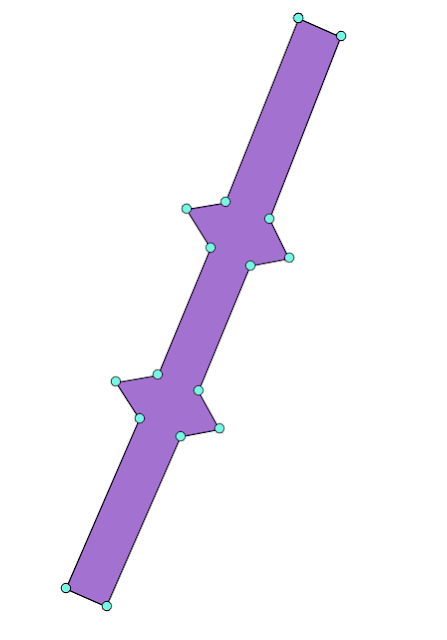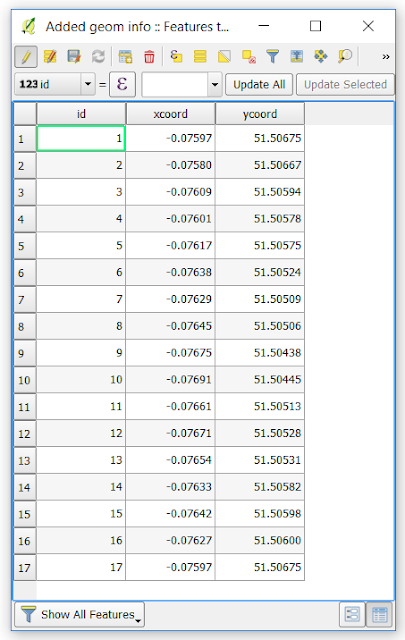### Geometry Objects in SQL Server using Latitude/Longitude coordinates

(2018-Mat-31) Support for spatial geometry functions was initially introduced in SQL Server 2008 and then it was greatly improved in Denali (SQL Server 2012) version of the product - https://docs.microsoft.com/en-us/sql/relational-databases/spatial/spatial-data-sql-server

Back then, when I was reading about the spatial features in SQL Server, I wondered where I could use this functionality with the creation of geo points, line polygons; however, working with geometry objects a bit more helped me to see some real use cases where this could be applicable.

Let's say you have the London Tower Bridge and you want to know when a taxi cab with your very important guest crosses this bridge. By creating a geo polygon for the bridge you can use spatial functions in SQL Server to check if a taxi cab GPS tracker coordinates intersect with the bridge polygon geometry object - STIntersects (geometry Data Type).First, I've located my map objects in the QGIS tool using Google Satelite layer:Then I created a polygon that would resemble the bridge area over the Thames River:Then by extracting the nodes of my polygon, I can see each individual geo points coordinates:And then after migrating this dataset into my dbo.LondonBridgeCoordinates SQL Server table, I can use this script to create the very same geometry object in my database:

```-- POLYGON definition
DECLARE @coords nvarchar(max);

-- POLYGON CREATION FROM LON/LAT COORDINATES
WITH polygon
AS (SELECT
id,
latitude,
longitude,
CONCAT(LTRIM(STR(longitude, 22, 6)), ' ', LTRIM(STR(latitude, 22, 6))) AS coords
FROM [dbo].[LondonBridgeCoordinates]),
-- LON/LAT CONCATENATION
polygon_coordinates
AS (SELECT
STUFF((SELECT
', ' + coords
FROM polygon p
ORDER BY p.id
FOR xml PATH (''), TYPE)
.value('(./text())', 'VARCHAR(MAX)'), 1, 2, '') AS polygon_coords,
(SELECT
CONCAT(LTRIM(STR(longitude, 22, 6)), ' ', LTRIM(STR(latitude, 22, 6))) AS coords
FROM [dbo].[LondonBridgeCoordinates]
WHERE id = 1)
AS first_coordinate)

SELECT  @coords = (polygon_coords) FROM polygon_coordinates
-- LET’S SEE HOW A POLYONG WOULD LOOK LIKE
SELECT  geometry ::STPolyFromText('POLYGON((' + @coords + '))', 4326).MakeValid()
```I can also save the output of the STPolyFromText function as a database object and use it with all other available spatial functions in SQL Server.

Please let me know if you can find other uses besides checking geo objects intersection. There are so many possibilities!